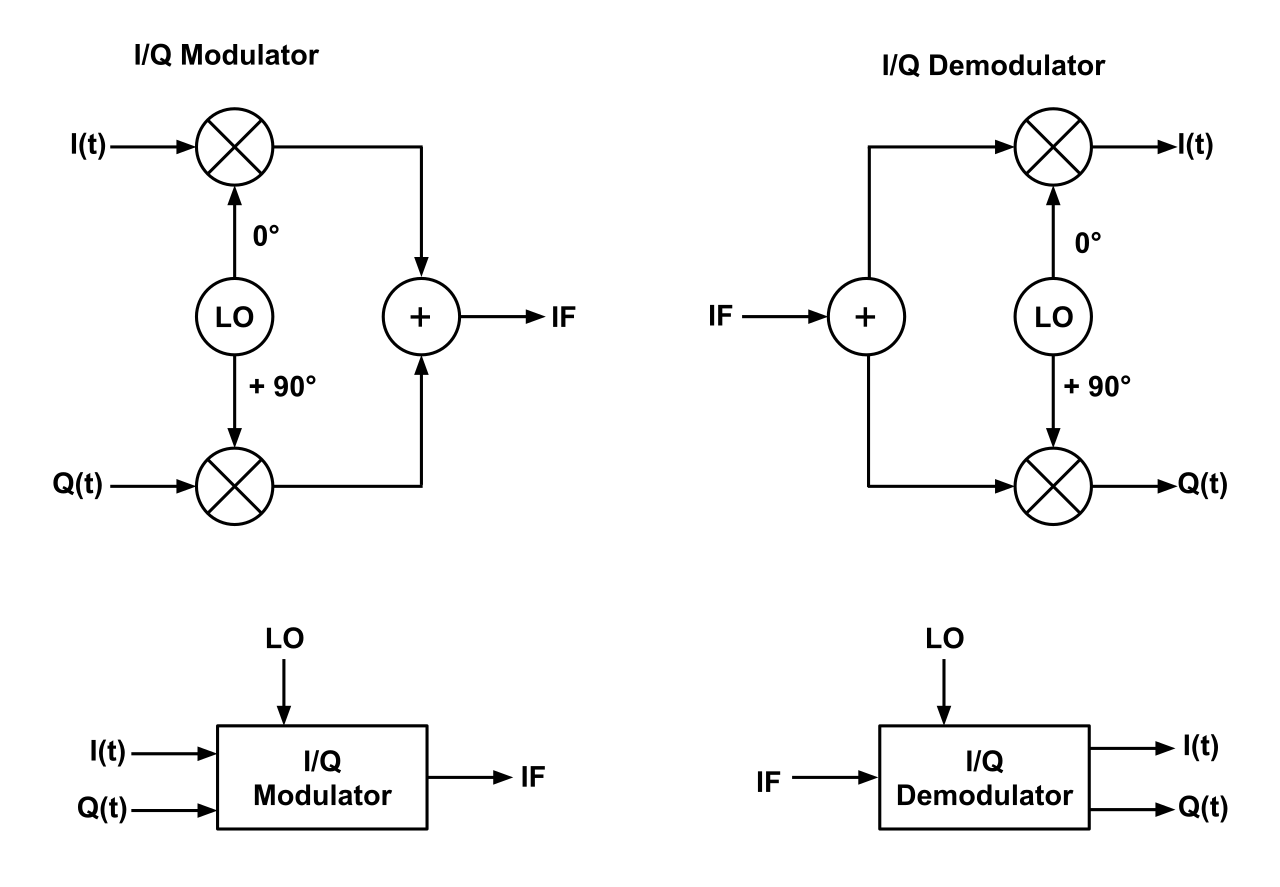# IQ Signals and IQ Modulation/Demodulation

The following application note provides an introduction to IQ signals and modulation.

## 1. On IQ Signals

A complex wave can be represented using two orthogonal value pairs, in the form of IQ signals. These two orthogonal numbers represent a complex waveform with a 90° phase relationship. The $I$ stands for “in-phase” and $Q$ stands for “quadrature”. In fact, a consine and sine wave are quadrature waveforms of each other.

We can say mathematically that our quadrature signal is represented as $I*cos(2\pi f t)$ and $Q*sin(2\pi f t)$, where by convention, the $I$ is the amplitude of the in-phase and $Q$ is the amplitude of the quadrature components. Figure 1 below shows this:

##### Figure 1: Basic IQ Signal (With I=Q=1)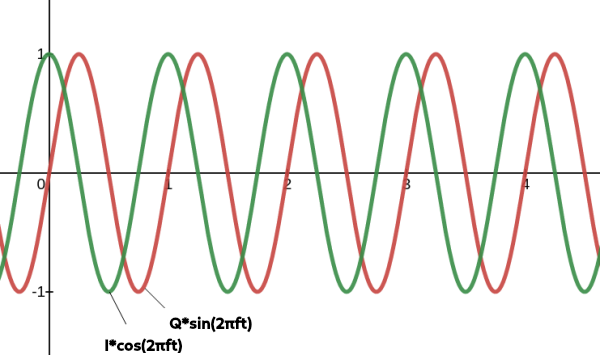### 1.1 Basics of IQ Modulation/Demodulation

The key to IQ modulation and demodulation is how we add quadrature signals for various modulation schemes. The block diagram below shows how adding $I$ and $Q$ works;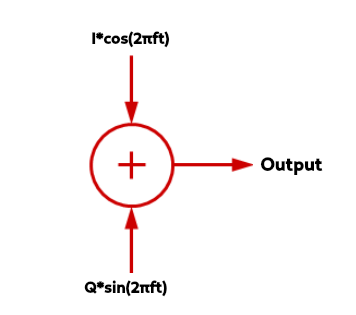As an example, by simply adding the I and Q values together, by superposition, if we were to say $I=1$ and $Q=1$, then we’d have a new waveform in black shown below:

##### Figure 3: Adding the I=1 and Q=1 Signal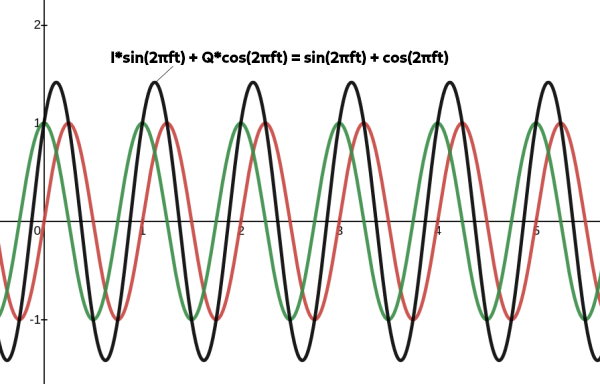##### Figure 3: Adding the I=1 and Q=3 Signal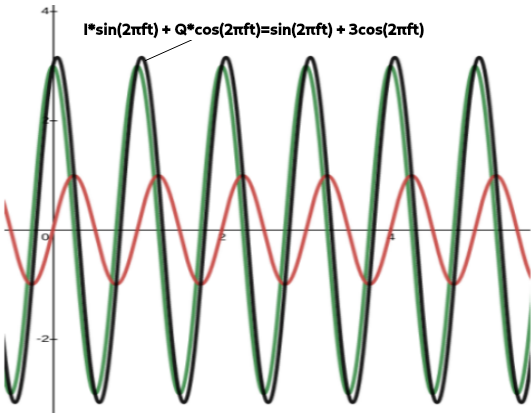In general, if $I$ or $Q$ changes or varies, the result are changes in amplitude, phase and frequency modulation of the sum. Essentially as the $I$ and $Q$ vectors change, the magnitude of the vector sum changes. The GIF below shows how this works using a phasor diagram– another way to visualize IQ signals:

##### Figure 5: How Adding I (x-axis) and Q (y-axis) Changes with a Phasor Diagram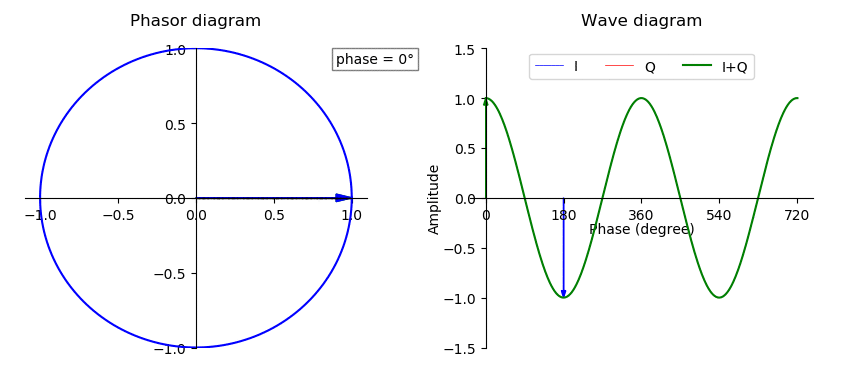Now, knowing how the complex plane works, and making our IQ signal a function of time, we can see that IQ pairs can be represented by a famous formula, Euler’s formula:

${e}^{i2\pi f t}= cos(2\pi f t) + isin(2 \pi f t)$

$= I(t) + iQ(t)$

And thus, we say the amplitude vector’s magnitude is given by:

$A= \sqrt{(I^2 + Q^2)}$

And the angle $\theta$ between the I vector and the A vector is:

$\theta= tan^{-1}(Q/I)$

### 1.2 IQ Modulators/Demodulators

To control how the $I$ and $Q$ signals are modulated/demodulated, the use of a IQ modulator and IQ demodulator is needed, which essentially mixes an LO frequency from 0-90° with the $I(t)$ and $Q(t)$ signals, as shown in the following block diagrams:

##### Figure 6: IQ Modulator and Demodulator